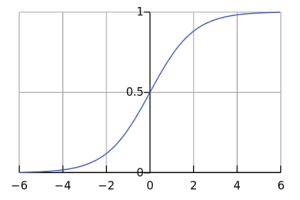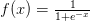Logistic regression is a classification model that uses input variables to predict a categorical outcome variable that can take on one of a limited set of class values. A binomial logistic regression is limited to two binary output categories while a multinomial logistic regression allows for more than two classes. Examples of logistic regression include classifying a binary condition as “healthy” / “not healthy”, or an image as “bicycle” / “train” / “car” / “truck”. Logistic regression applies the logistic sigmoid function to weighted input values to generate a prediction of the data class.Figure: The logistic sigmoid function. Image Source

A logistic regression model estimates the probability of a dependent variable as a function of independent variables. The dependent variable is the output that we are trying to predict while the independent variables or explanatory variables are the factors that we feel could influence the output. Multiple regression refers to regression analysis with two or more independent variables. Multivariate regression on the other hand refers to regression analysis with two or more dependent variables.

### Linear and Logistic Regression

Linear regression is the more common form of regression and fits a linear model through a set of data points. The output y can be estimated as a straight line that can be visualized as

y = mixi + c + E

xi are the input variables, and the parameters mi, c, and E are the regression coefficients, the constant offset, and the error respectively. The coefficients mi can be interpreted as the increase in a dependent variable for a unit increase in the respective independent variable. The parameters in an ordinary linear regression model are linear, and both the input variables (independent variables) and output variable (dependent variable) are normally distributed with constant variance.

A generalized linear regression does not need the data input to have a normal distribution. The test data can have any distribution. Logistic regression is a special case of the generalized linear regression where the response variable follows the logit function.

The input of the logit function is a probability p, between 0 and 1. The odds ratio for probability p is defined as p/(1-p), and the logit function is defined as the logarithm of the Odds ratio or log-odds.

Logit(p) = Log(odds) = Log (p/(1-p))

### Goodness-of-fit of a Logistic Regression

The quality of a logistic regression model is determined by measures of fit and predictive power. R-squared is a measure of how well the independent variable in the logistic function can be predicted from the dependent variables, and ranges from 0 to 1. Many different ways exist to calculate R-square including the Cox-Snell R2 and the McFadden R2. Goodness of fit, on the other hand, can be measured using tests such as the Pearson chi-square, Hosmer-Lemeshow, and Stukel tests. The right type of test to use will depend on factors such as the distribution of p-values, interactions and quadratic effects, and grouping of data.

### Applications of Logistic Regression

Logistic regression is similar to a non-linear perceptron or a neural network without hidden layers. Logistic regression is most valuable for areas where data are scarce, like the medical and social sciences where it is used to analyze and interpret results from experiments. Because regression is simple and fast, it is also used for very large data sets. Regression, however, cannot be applied to predict continuous outcomes or used with data sets that are not independent. There is also a possibility of overfitting the model to the data when using logistic regression.

### Logistic Regression in Deep Learning

In deep learning, the last layer of a neural network used for classification can often be interpreted as a logistic regression. In this context, one can see a deep learning algorithm as multiple feature learning stages, which then pass their features into a logistic regression that classifies an input. Adapting these deep learning models to execute on the GPU can lead to significant increases in performance. The NVIDIA Deep Learning SDK provides powerful tools and libraries for designing and deploying GPU-accelerated deep learning applications including logistic regression. The Deep Learning SDK requires CUDA Toolkit, which offers a comprehensive development environment for building GPU-accelerated deep learning algorithms.